# 无需学习Python，一个公式搞定领导想看的大屏

07/19 08:58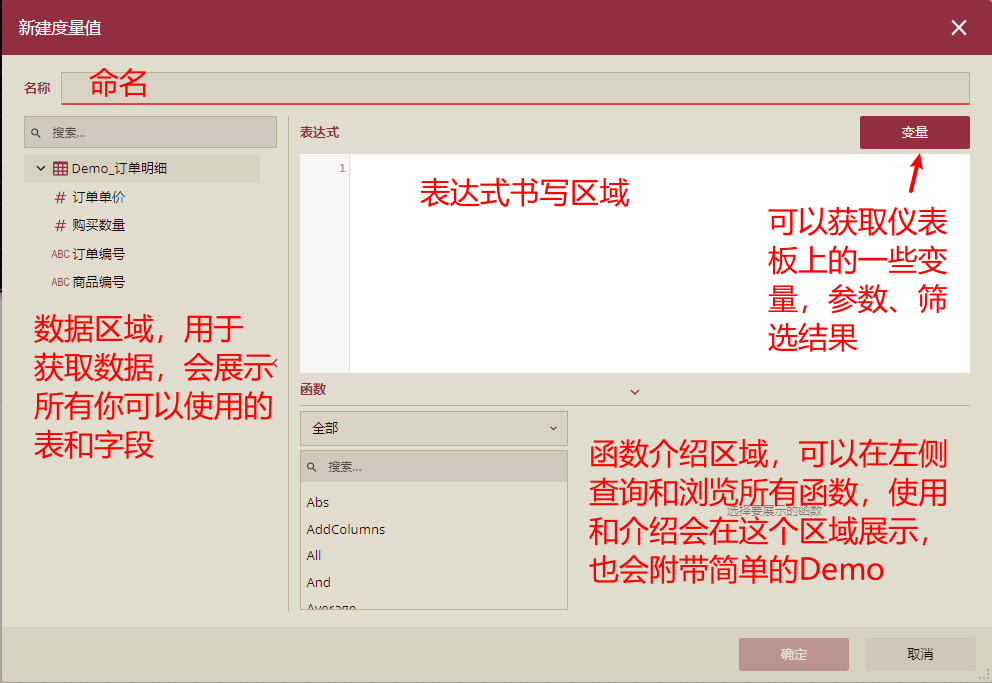1）在书写表达式的时候，通常可以将比较困难的表达式拆分为多个表达式。例如(a+b)*c,我们可以先将a+b计算的结果作为一个新的计算列，然后这个计算列会作为一个新的字段被再次复用去乘c，这样就可以抽取共享字段，提高复用性。

2）合理利用Filter、calculate等过滤函数，可以一定程度加快表达式的计算，当不再需要某些值时，减少过多的if使用，而是直接将这些数据过滤掉，提高表达式速度。

3）在使用的过程中 ’ ’ 单引号中间是写“表名”、 []中括号中间写“字段名”、 “”双引号代表“字符串”。例如: ’销售明细’[订单金额] 代表的是销售明细表的订单金额字段，在多个表没有字段名重复的时候，可以直接使用[]引用字段，不带表名。(注意：所有都是英文字符)

1. 同比-环比

var basetable = SelectColumns(

'同比测试',

"年度", '同比测试'[下年度],

"订单金额",'同比测试'[订单金额])

return Calculate(

SumX(basetable,[订单金额]), Values([年度]))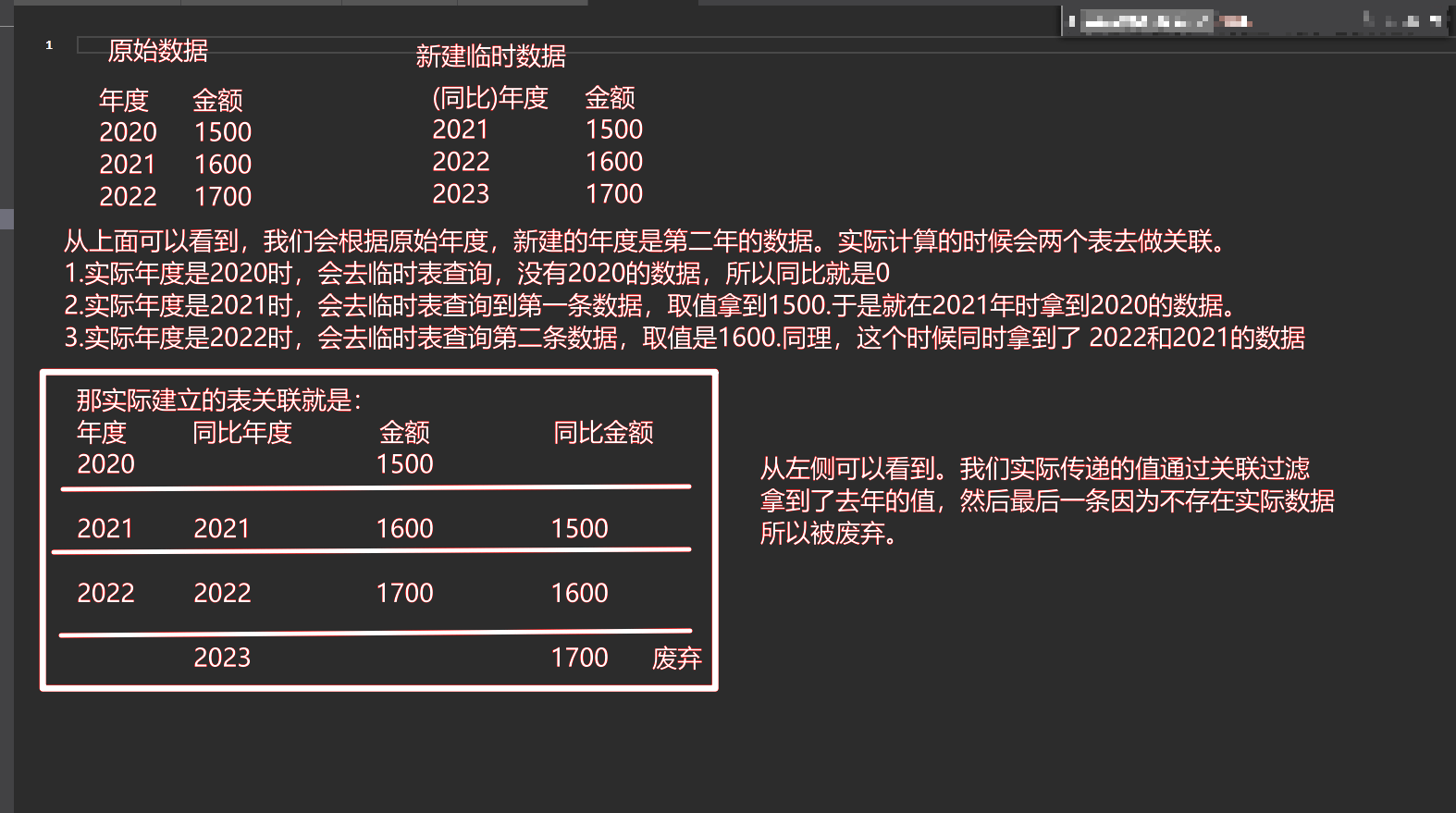Divide('同比测试'[当前年合计] - '同比测试'[去年合计], '同比测试'[去年合计])

var basetable = SelectColumns(

'同比测试',

"年度", '同比测试'[环比年度],

"季度", '同比测试'[环比季度],

"订单金额",'同比测试'[订单金额])

return Calculate(

SumX(basetable,[订单金额]), Values([年度]))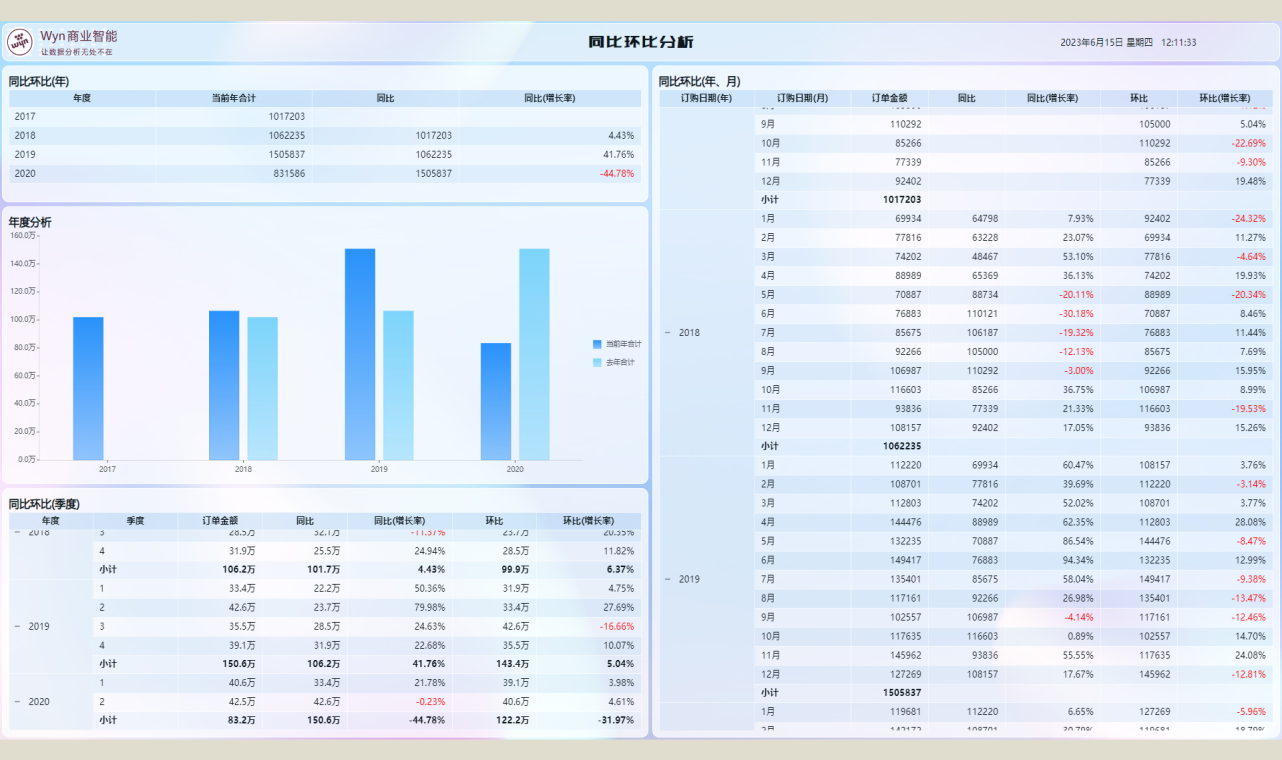1. 区间分析

var sales = SUMX('订单明细','订单明细'[购买数量]\*'订单明细'[订单单价])

var sales1 =

calculate(sales,Values('销售大区'[大区]))

var categories=

SWITCH(

TRUE,

sales1 \< 8000000, "小于800万",

sales1 \>= 8000000 && sales1 \< 12000000, "大于800万小于1200万",

sales1 \>= 12000000 , "大于1200万"

)

return categories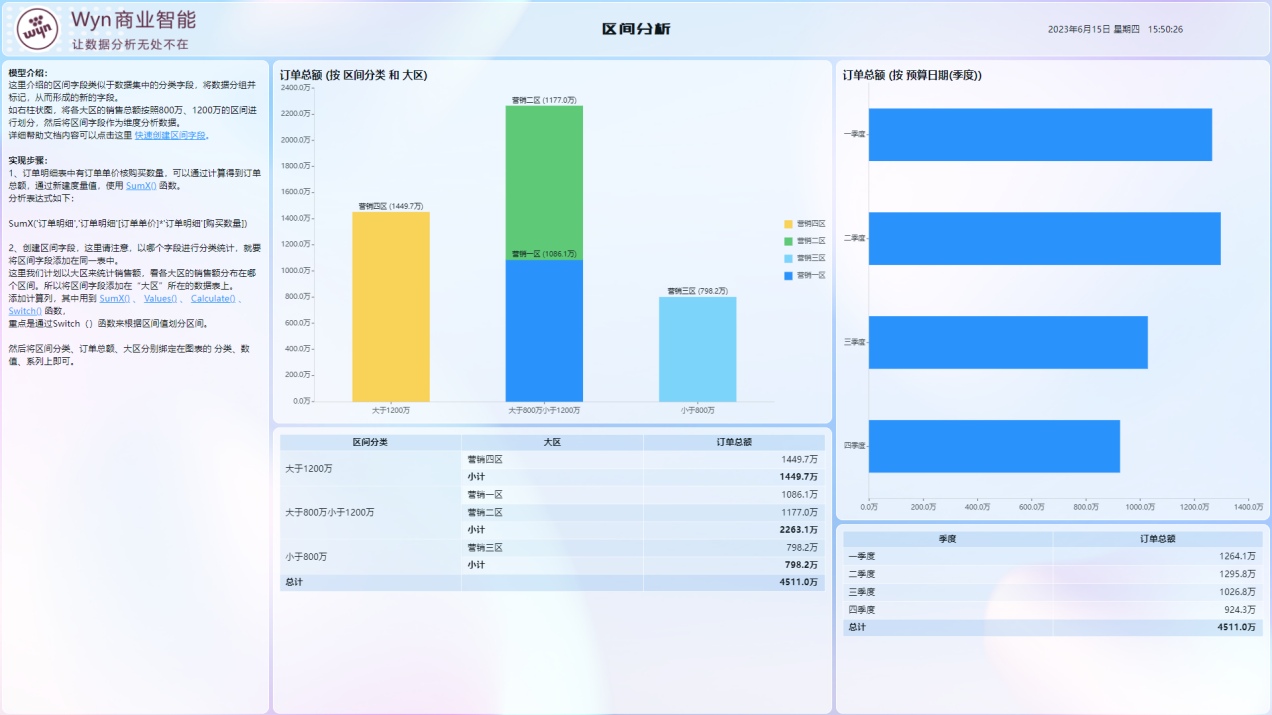1. RFM模型分析

• R (Recency),最近一次消费时间间隔
• F (Frequency),消费频率
• M (Monetary),消费金额

R_Avg

CALCULATE(

AVERAGEX(

SUMMARIZECOLUMNS(

'Customer'[Name],

"rdayValues",

datediff(

'Sales'[Rday],

'Sales'[Rmax],

day

)

),

[rdayValues]

),

REMOVEFILTERS('Customer'[Name])

)


F_Avg

CALCULATE(

AVERAGEX(

SUMMARIZECOLUMNS(

'Customer'[Name],

"fCount",

calculate(

DISTINCTCOUNTX('Sales','Sales'[Order Number])

)

),

[fCount]

),

REMOVEFILTERS('Customer'[Name])

)


M_Avg

CALCULATE(

AVERAGEX(

SUMMARIZECOLUMNS(

'Customer'[Name],

"mValues",

calculate(

'Sales'[M]

)

),

[mValues]

),

REMOVEFILTERS('Customer'[Name])

)


R：DATEDIFF( 'Sales'[Rday], 'Sales'[Rmax], Day )

F：DISTINCTCOUNTX('Sales','Sales'[Order Number])

M：SUMX( 'Sales', 'Sales'[Quantity]\*'Sales'[Net Price] )


R↑F↑M↑：重要价值客户

R↑F↑M↓：一般价值客户

R↑F↓M↑：重要发展客户

R↑F↓M↓：一般发展客户

R↓F↑M↑：重要保持客户

R↓F↑M↓：一般保持客户

R↓F↓M↑：重要挽留客户

R↓F↓M↓：一般挽留客户

R：IF( 'Sales'[R]\<='Sales'[Ravg], "R_UP", "R_DOWN" )

F:：IF( 'Sales'[F]\<='Sales'[Favg], "F_UP", "F_DOWN" )

M：IF( 'Sales'[M]\<='Sales'[Mavg], "M_UP", "M_DOWN" )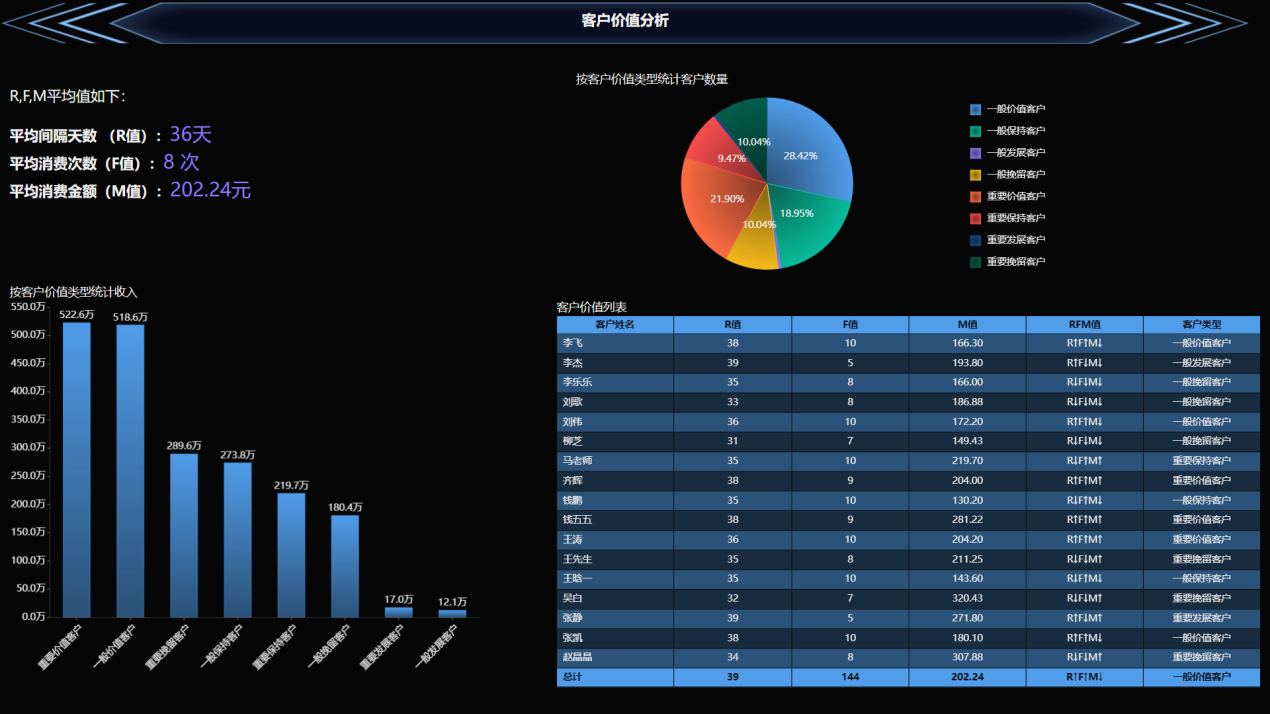Wyn商业智能软件除了在BI设计层面可以做表达式计算，在数据获取层面也可以做表达式计算、分组计算以及权限控制等完整的功能。作为一款B/S架构的软件，客户只需要能访问服务器即可做出属于自己设计和展示的大屏。

Spring Boot框架下实现Excel服务端导入导出

Svelte 框架结合 SpreadJS 实现纯前端类 Excel 在线报表设计1 评论
0 收藏
0